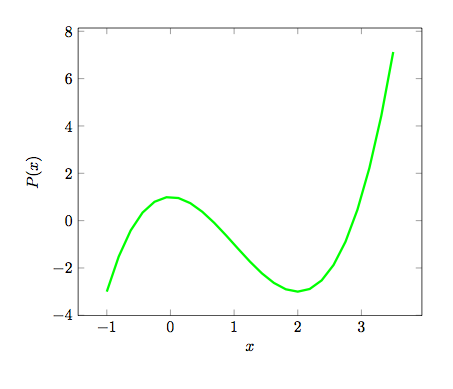# A strange equation II

Algebra Level 5The three real roots of the polynomial $P(x)=x^{3}-3x^{2}+1$ can be expressed as:

$x_{1}=-b\cos(e°)+a , x_{2}=-c\cos(f°)+a, x_{3}=d\cos(g°)+a,$

where $x_{1}, $0°, $0°, $0°, such that $a$, $b$, $c$, $d$, $e$, $f$ and $g$ are positive integers; and $e$, $f$ and $g$ are integer degrees. Find $a+b+c+d+e+f+g$.

You may also try Part III.

×

Problem Loading...

Note Loading...

Set Loading...